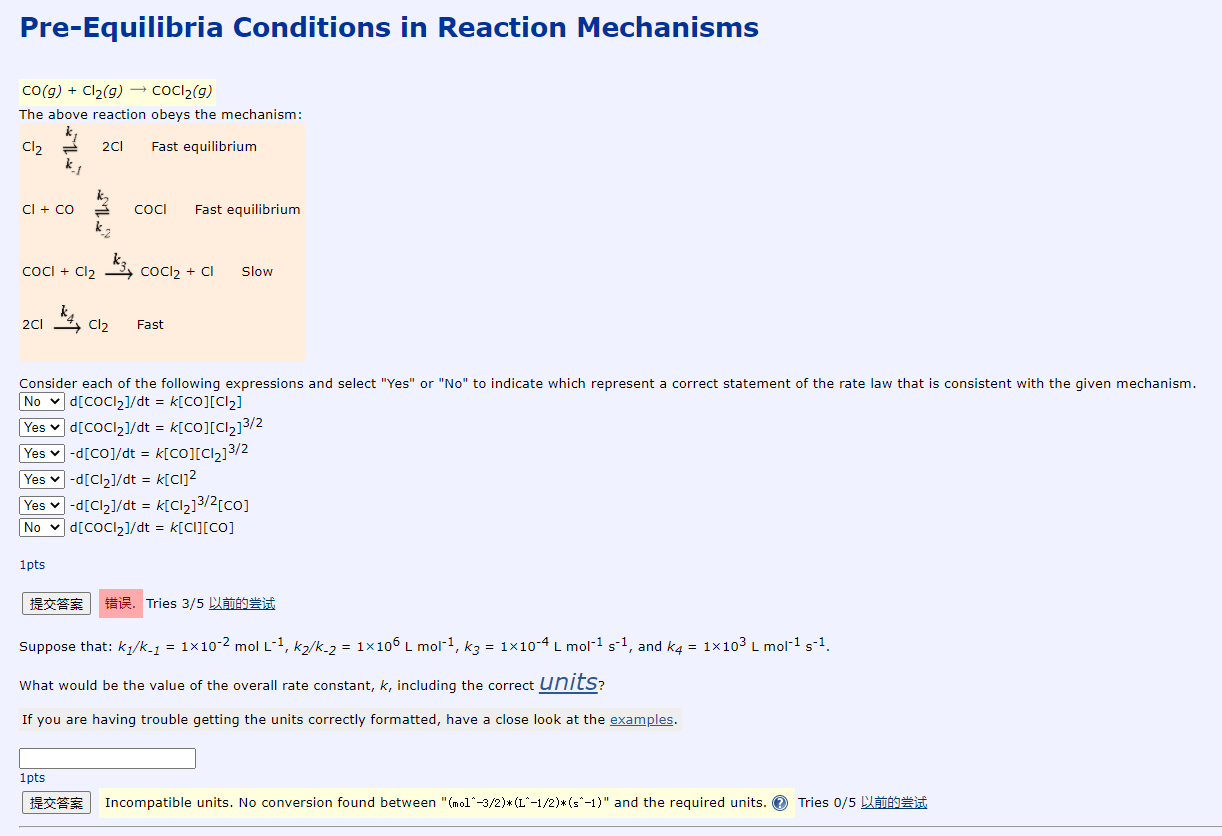# Question Solved1 AnswerPre-Equilibria Conditions in Reaction Mechanisms CO(g) + Cl2(g) → COC12(9) The above reaction obeys the mechanism: Cl2 2C1 Fast equilibrium Cl + CO COCI Fast equilibrium COCl + Cl2 COCl2 + CI Slow 2cl Cl2 Fast Consider each of the following expressions and select "Yes" or "No" to indicate which represent a correct statement of the rate law that is consistent with the given mechanism. No d[CoCl2]/dt = K[CO](C12] Yes v d[COC12]/dt = k[CO][C12]3/2 Yes v -d[Co]/dt = k[CO][C12]3/2 Yes -d[Cl2]/dt = k[CI]2 Yes - d[Cl2]/dt = k[C12]3/2[Co] No d[CoCl2]/dt = k[Cl][CO] 1pts 提交答案 错误, Tries 35 以前的尝试 Suppose that: kz/k-1 = 1x10-2 mol L-1, kz/k-2 = 1*106 L mol-1, k3 = 1*10-4 L mol-1 5-1, and k4 = 1x103 L mol-1 5-1. What would be the value of the overall rate constant, k, including the correct units? If you are having trouble getting the units correctly formatted, have a close look at the examples. 1pts 提交答案 Incompatible units. No conversion found between "molº-3/2)*(L"-1/2)*(sº-1)" and the required units. Tries 0/5 DLHŠITranscribed Image Text: Pre-Equilibria Conditions in Reaction Mechanisms CO(g) + Cl2(g) → COC12(9) The above reaction obeys the mechanism: Cl2 2C1 Fast equilibrium Cl + CO COCI Fast equilibrium COCl + Cl2 COCl2 + CI Slow 2cl Cl2 Fast Consider each of the following expressions and select "Yes" or "No" to indicate which represent a correct statement of the rate law that is consistent with the given mechanism. No d[CoCl2]/dt = K[CO](C12] Yes v d[COC12]/dt = k[CO][C12]3/2 Yes v -d[Co]/dt = k[CO][C12]3/2 Yes -d[Cl2]/dt = k[CI]2 Yes - d[Cl2]/dt = k[C12]3/2[Co] No d[CoCl2]/dt = k[Cl][CO] 1pts 提交答案 错误, Tries 35 以前的尝试 Suppose that: kz/k-1 = 1x10-2 mol L-1, kz/k-2 = 1*106 L mol-1, k3 = 1*10-4 L mol-1 5-1, and k4 = 1x103 L mol-1 5-1. What would be the value of the overall rate constant, k, including the correct units? If you are having trouble getting the units correctly formatted, have a close look at the examples. 1pts 提交答案 Incompatible units. No conversion found between "molº-3/2)*(L"-1/2)*(sº-1)" and the required units. Tries 0/5 DLHŠI
More
Transcribed Image Text: Pre-Equilibria Conditions in Reaction Mechanisms CO(g) + Cl2(g) → COC12(9) The above reaction obeys the mechanism: Cl2 2C1 Fast equilibrium Cl + CO COCI Fast equilibrium COCl + Cl2 COCl2 + CI Slow 2cl Cl2 Fast Consider each of the following expressions and select "Yes" or "No" to indicate which represent a correct statement of the rate law that is consistent with the given mechanism. No d[CoCl2]/dt = K[CO](C12] Yes v d[COC12]/dt = k[CO][C12]3/2 Yes v -d[Co]/dt = k[CO][C12]3/2 Yes -d[Cl2]/dt = k[CI]2 Yes - d[Cl2]/dt = k[C12]3/2[Co] No d[CoCl2]/dt = k[Cl][CO] 1pts 提交答案 错误, Tries 35 以前的尝试 Suppose that: kz/k-1 = 1x10-2 mol L-1, kz/k-2 = 1*106 L mol-1, k3 = 1*10-4 L mol-1 5-1, and k4 = 1x103 L mol-1 5-1. What would be the value of the overall rate constant, k, including the correct units? If you are having trouble getting the units correctly formatted, have a close look at the examples. 1pts 提交答案 Incompatible units. No conversion found between "molº-3/2)*(L"-1/2)*(sº-1)" and the required units. Tries 0/5 DLHŠI